# Viet's relations

## Relations between roots and coefficients of algebraic equations

Viet's relations are relations between coefficients and roots of algebraic equations.

If we have an algebraic equation rank n (n ≥ 1),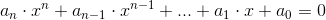with an ≠ 0 and a0, ..., an complex numbers

and if x1, x2, ..., xn are its roots, then we have the following relations: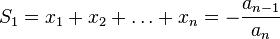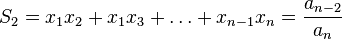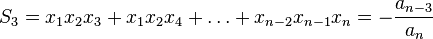......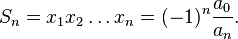These are called Vièt's relations and they are relations between the coefficients and the roots of an algebraic equation

They were established, as their name says, by the French mathematician François Viète.

## Special case: second-degree equation

Let be the second-degree equation: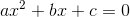,

where a ≠ 0.

The Discriminant (Δ) are calculated with this formula:If Δ 0, then we have real solutions, x1 and x2 .

At this case the relations of Viète are: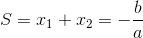Keywords: algebra, algebraic equations, relationships of roots, the equation of the nth degree, second degree equation

### Forum

Here you can discuss about mathematic, about algebra, geometry, trigonometry.

It is not mandatory to be logged in on this forum but it is nice to have an account. You can ask about mathematics just with your name and your email.

This maths forum is one of the easiest forums to use it.

>> Go to Math Forum